Importance: Medium ✭✭
 Author(s): Makowsky, Janos A.
 Subject: Logic » Finite Model Theory
 Keywords: Blatter-Specker Theorem FMT00-Luminy
 Recomm. for undergrads: no
 Posted by: dberwanger on: May 18th, 2012

Let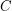be a class of finite relational structures. We denote by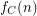the number of structures inover the labeled set. For any classdefinable in monadic second-order logic with unary and binary relation symbols, Specker and Blatter showed that, for every, the functionis ultimately periodic modulo.

Question   Does the Blatter-Specker Theorem hold for ternary relations.

Our exposition follows closely [BS84].

## Counting labeled structures moduloLetbe a class of finite structures for one binary relation symbol. We define for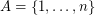#### Examples:

\item Ifconsists of all-structures,. \item Ifconsists of bijections,\item Ifis the class of all (undirected, simple) graphs,. \item If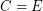is the class of all equivalence relations, then, the {\em Bell Numbers}. \item Ifis the class of all equivalence relations with two classes only, of the same size,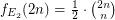. Clearly,. \item If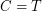is the class of all trees,, {\em Caley}.

We observe the following:And for eachthe functions,,,are ultimately periodic.

However,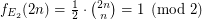iff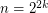, hence is not periodic.

## Monadic second-order logic definable classes

The first four examples (all relations, all bijections, all graphs, all equivalence relations) are definable in First Order Logic. The trees are definable in Monadic Second Order Logic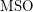..is definable in Second Order Logic, but it is not-definable. If we expandto have the bijection between the classes we get structures with two binary relations. The class is now-definable. Let us denote the corresponding counting function. We haveforlarge enough.

## Periodicity and linear recurrence relations

The periodicity ofis usually established by exhibiting a linear recurrence relation:

There exists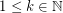and integers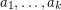such that for all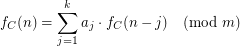#### Examples.

\item In the case ofwe have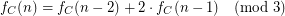\item In the case of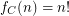we have for all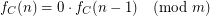In this case we say thattrivializes.

## The Blatter-Specker Theorem

Theorem  (BS84)   Letbe a binary vocabulary, i.e. all relation symbols are at most binary. Ifis a class of finite-structures which is-definable, then for allis ultimately periodic.

Moreover, there existsand integerssuch that for alli.e we have a linear recurrence relation.

In [F03] Fischer showed that the Specker-Blatter Theorem does not hold for quaternary relations.

The case of ternary relations remains open.

See also [FM06] for further developments on the topic.

## Bibliography

[BS84]* C. Blatter and E. Specker, Recurrence relations for the number of labeled structures on a finite set, Logic and Machines: Decision Problems and Complexity, E. Börger, G. Hasenjaeger and D. Rödding, eds, LNCS 171 (1984) pp. 43-61.

[F03] E. Fischer, The Specker-Blatter theorem does not hold for quaternary relations, Journal of Combinatorial Theory Series A 103(2003), 121-136.

[FM06] E. Fischer and J. A. Makowsky, The Specker-Blatter Theorem revisited: Generating functions for definable classes of stuctures. In Computing and Combinatorics (COCOON 2003) Proc., LNCS vol. 2697 (2003), 90-101.

[S88] E. Specker, Application of Logic and Combinatorics to Enumeration Problems, Trends in Theoretical Computer Science, E. Börger ed., Computer Science Press, 1988, pp. 141-169. Reprinted in: Ernst Specker, Selecta, Birkhäuser 1990, pp. 324-350.

* indicates original appearance(s) of problem.

## Reply

More information about formatting options